# Matrices for Tech Artists, a Cheat Sheet

## Think of Matrices as Spaces

If you have a vector, it spans a space. For just a vector, this space is a line. How do you know that it does? You can get every point in that space just using the vector, and a scalar (a number) you multiply it with. For example the global right vector (1, 0, 0). You could get every point on that line by multiplying a number with that vector. For example the point (2, 0, 0) is 2*(1, 0, 0).

## Identity Matrix

Identity Matrix is the basis for everything. The space its columns span is simply your world coordinate, your standard cartesian coordinate, your forward, up right. Name it what you want. This matrix is important, because by understanding it and comparing other matrices with it, you know how the space of that matrix is different compared to your standard space. The same way that by comparing the number 5 to number 1, you know 5 is 5 times bigger than 1.

## Uniform Scaled Matrix

If you change numbers on the main diagonal of the matrix, you are scaling the x, y, z component of the vector you multiply with. In other words the space the columns span, is one where the axis are scaled compared to the Identity column space. In case of a mesh you are scaling the mesh. For example lets take 0.5 for all entries of the diagonal. Since we are changing all the diagonal entries the same, the mesh will be scaled uniformly.

## Axis Scaled Matrix

What if you only change one of the entries? Or change them independently? In that case you are scaling not uniformly in all axis, but scaling only along one axis. For example, lets take the following matrix. The column space of this matrix is scaled in x axis 3 times compared to the identity matrix. You can see that well if you compare the x axis in the picture (red arrow) compared to the other two.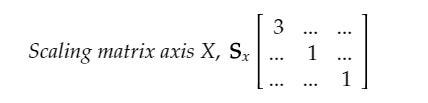Figure -7- Axis scaled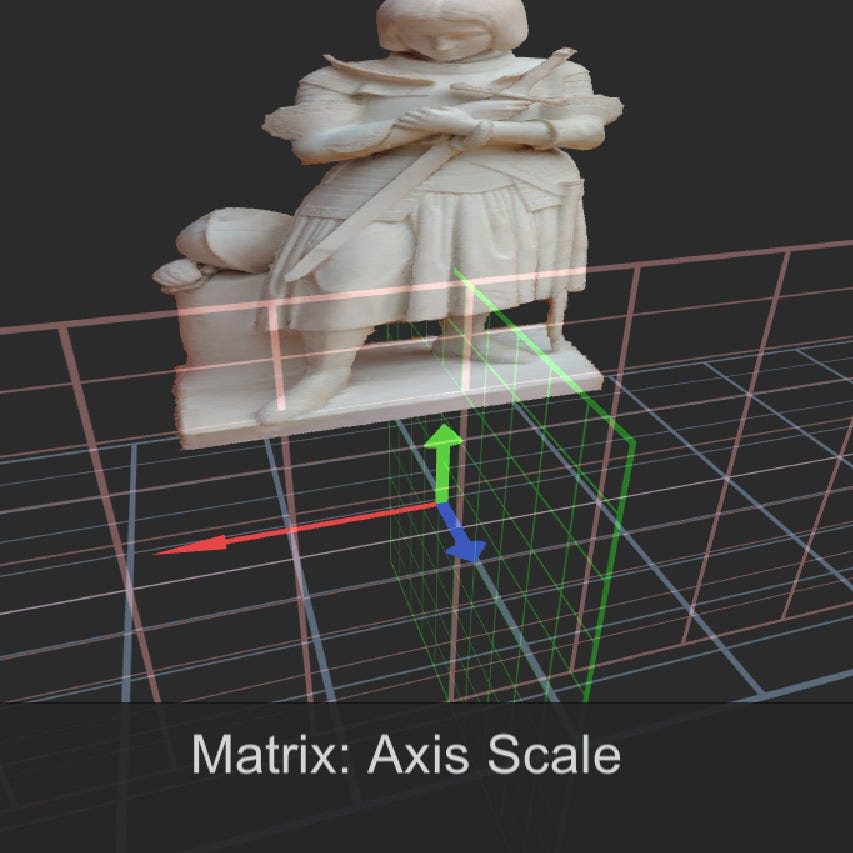Figure -8- Mesh scaled 3 times along an axis

## Mirror Matrix

You can also reflect or mirror your vector around an axis. This matrix’s column space is mirrored in a certain way compared to the identity. To do this you need to multiply an entry of the diagonal with minus.

## Matrix with Normalized Basis

So far we have only been modifying the diagonal and leaving everything else zero. Having all other entries as zero means, that when calculating the new entries of the transformed vector, the x component does not contribute to the calculation of the y or the z, or otherwise. If we start changing other entries, all sort of things will happen in one go. So I am going to break it further in different scenarios.

## Matrix with Orthogonal Basis

So what if the basis are not normalized but are orthogonal with respect to eachother?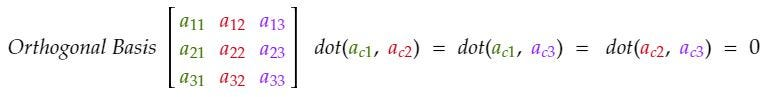Figure -15- matrix with orthogonal basis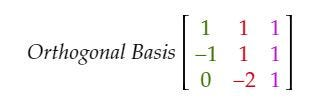Figure -16- matrix with orthogonal basis example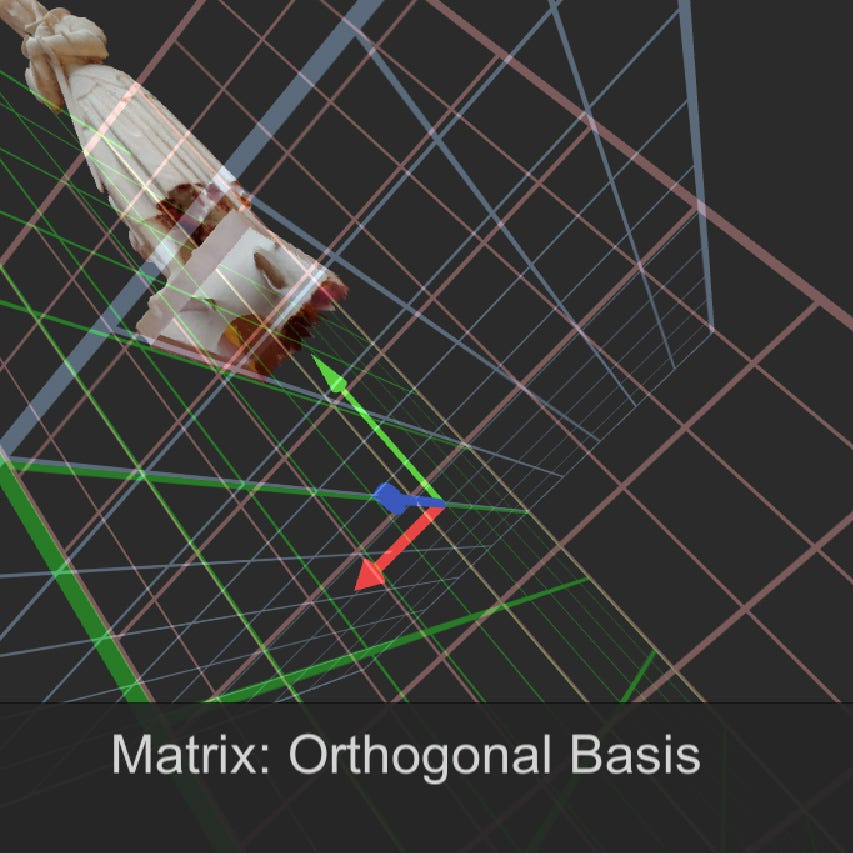Figure -17- mesh transformed with matrix with orthogonal basis

## Orthogonal Matrices

The column space of the matrix above was the identity matrix rotated and scaled, if we normalize the basis, we would take out the scaling. What we are left with is then just the rotation. This matrix should have been called an orthonormal matrix, but since mathematicians like pain and confusion, they named this matrix the orthogonal matrix.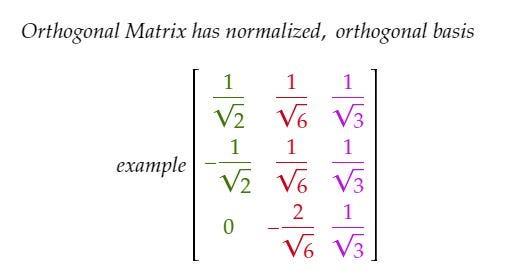Figure -18- Orthogonal matrix example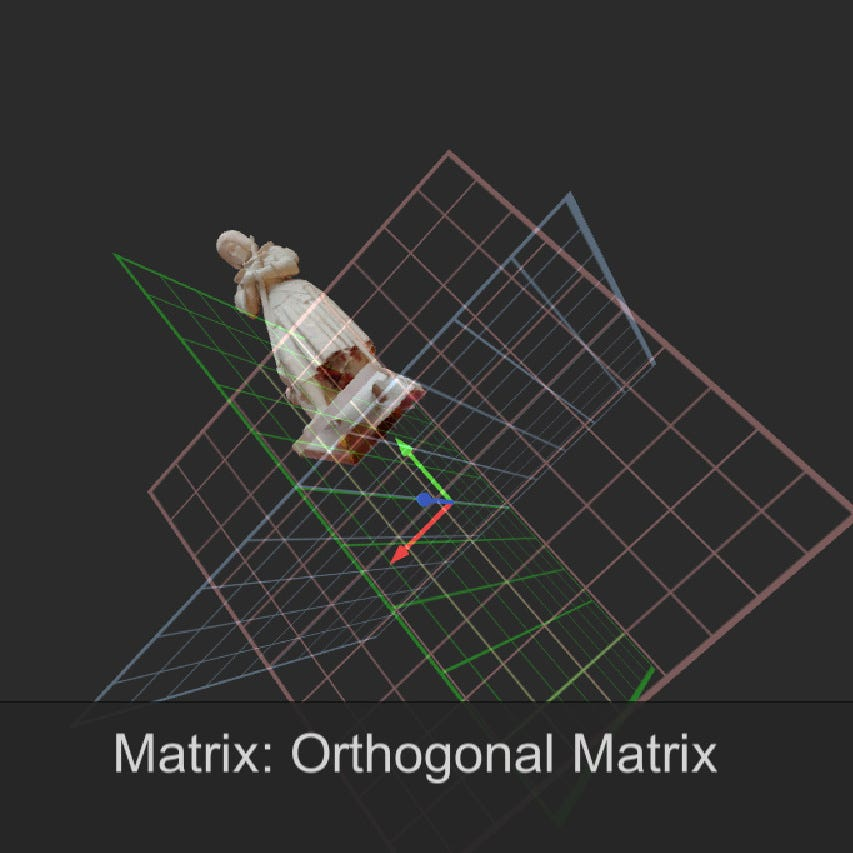Figure -19- mesh transformed by an orthogonal matrix

## Rotation Matrices

So we already derived a rotation matrix above, but there are also formulas for this, which let you rotate around x, y or z. Here are the formulas:

## What about Translation

You might have noticed that translation is missing. Well what we have been doing so far is a so called Linear Transformation. This group of transformations have a series of neat properties, but the relevant one is that they keep the zero at zero. None of the above matrices would move a vector (0,0,0) to be anything beside what it is. This obviously makes it impossible to have a translation, because every translation would break this rule.

## Homogeneous Coordinates

People are smart. They realized you can encode translation in your matrix multiplication, if you use a higher dimensional matrix than you need. Instead of a 3x3 matrix you would use a 4x4 matrix to transform a 3 dimensional point. You can’t multiply a 4x4 matrix with a 3 dimensional vector though. To fix this you convert your cartesian coordinates to a 4 dimensional homogeneous coordinates. You can always switch between the two coordinate systems by following simple rules. For example the new component you add to your matrix, the w component, should be 1 if you wish to transform a point, and 0 if you wish to transform a direction (this practically turns off translation, since directions are unbound vectors and don’t have a fix origin). Another one is once you are done with your matrix multiplication, divide your xyz components of the vector with w component to normalize the coordinates back to Cartesian. In rendering this is typically referred to as perspective divide, since this is where the effect of perspective is applied. The frustum is skewed from a homogeneous unit cube, which is convenient for clipping, back to a pyramid.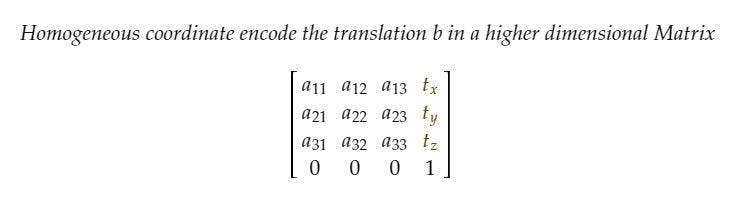Figure -24- Homogeneous matrix coordinate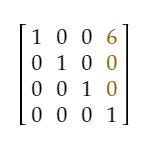Figure -25- Translation along x axis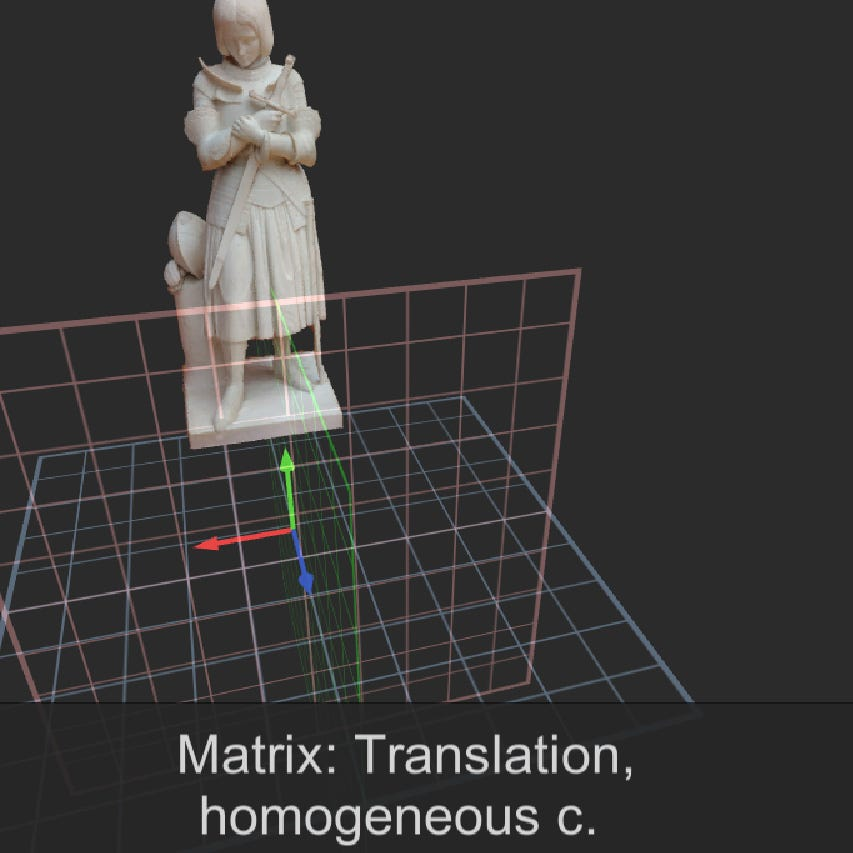Figure -26- Translation in X axis with 6 units

## Projection Matrices

These feel like magic. Though they are not. They do the exact same thing as what we have discussed so far. They transform the point in a new space, with a very specific set of criterias. This is a non affine transformation since the xyz component are scaled as a function of their distance from the near plane of the camera.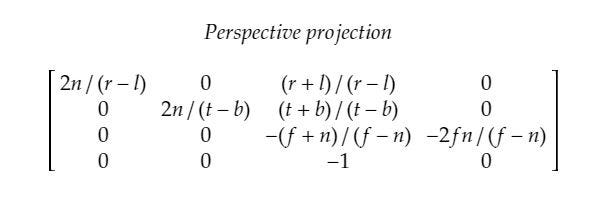Figure -27- Projection Matrix. n=near, f=far, t=top, r=right, l=left, b=bottom

## Some Extra Details

Here are some random stuff I would like to add on top.